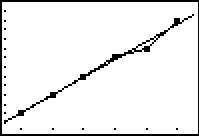# Knowledge Base

## Solution 27210: Creating and Viewing a Residual List on the TI-83 Plus family of graphing calculators.

### How do I view a residual list on the TI-83 Plus family of graphing calculators?

When the TI-83 Plus family of graphing calculators calculates a regression, there will be a difference between the values of f(x) as calculated and the value as determined by the "best fitting" line. This difference is called a residual.

When regressions are calculated, these residuals are automatically stored within a list called RESID. To view the RESID list, place it into a list in the List editor.

For example, calculate a linear regression for the following values:

L1: {1, 2, 3, 4, 5, 6} L2: {2, 4, 6, 8, 9, 12}

• Press [STAT] .
• Press [↑] until cursor is on top line, highlighting L1.
• Press [CLEAR] [ENTER].
• Press [↓]  [↓]  [↓]  [↓]  [↓]  [↓]  [↓].
• Press [→] [↑] [↑] [↑] [↑] [↑] [↑] [↑].
• Press [CLEAR] [ENTER].
• Press [↓]  [↓]  [↓]  [↓]  [↓]  [↓]  [↓].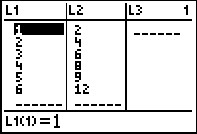• Press [2ND] [QUIT]
• Press [STAT] [→].
• Choose [4:LinReg (ax+b)].
• Press [2nd] [L1] [,] [2nd] [L2] [,] [VARS] [→]   [ENTER].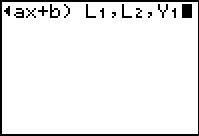• The linear regression will now be calculated and stored to Y1.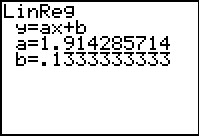To view the RESID list:

• Press [STAT] .
• Press [→] until you get to L6.
• Press [↑] [→].
• Press [2nd] [LIST] to open the List Editor
• Press [↓] until you reach the listname RESID then press [ENTER].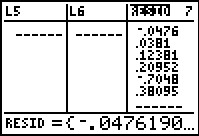To graph the linear regression along with the statistical data:

• Press [2nd] [STAT PLOT] .
• Use the settings listed in the image below. To change the menu settings use the arrow keys to navigate the options and press the [Enter] key to select an option.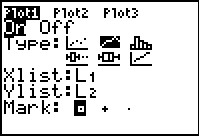• Press [ZOOM] .